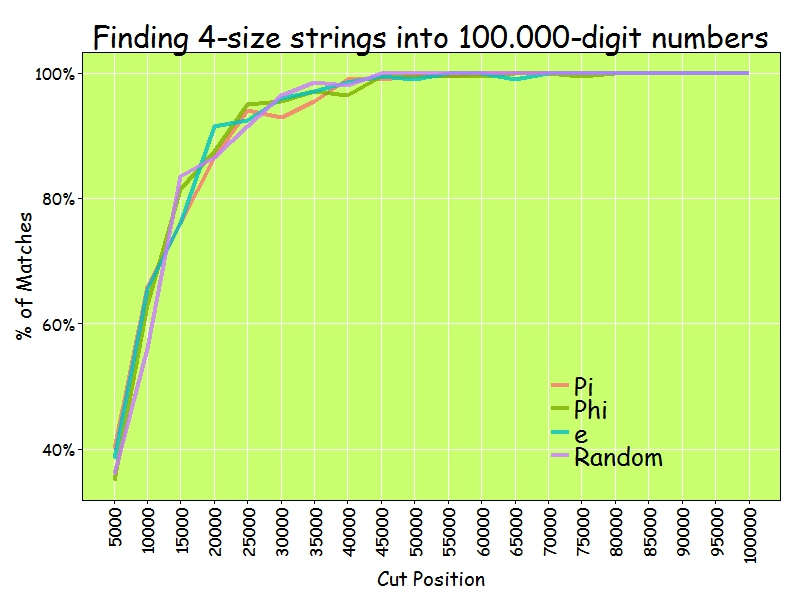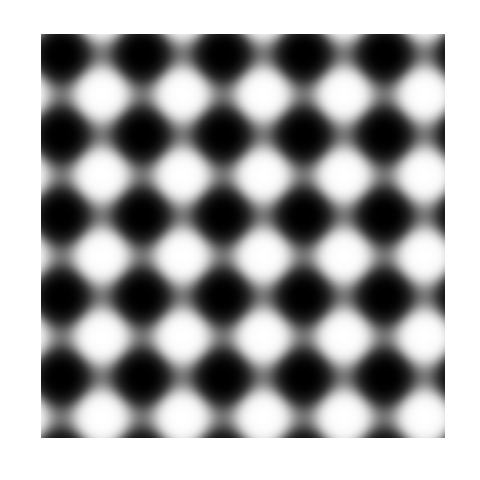# The World We Live In #5: Calories And Kilograms

I enjoy doing new tunes; it gives me a little bit to perk up, to pay a little bit more attention (Earl Scruggs, American musician)

I recently finished reading The Signal and the Noise, a book by Nate Silver, creator of the also famous FiveThirtyEight blog. The book is a very good reading for all data science professionals, and is a must in particular for all those who work trying to predict the future. The book praises the bayesian way of thinking as the best way to face and modify predictions and criticizes rigid ways of thinking with many examples of disastrous predictions. I enjoyed a lot the chapter dedicated to chess and how Deep Blue finally took over Kasparov. In a nutshell: I strongly recommend it.
One of the plots of Silver’s book present a case of false negative showing the relationship between obesity and calorie consumption across the world countries. The plot shows that there is no evidence of a connection between both variables. Since it seemed very strange to me, I decided to reproduce the plot by myself.

I compared these two variables:

• Dietary Energy Consumption (kcal/person/day) estimated by the FAO Food Balance Sheets.
• Prevalence of Obesity as percentage of defined population with a body mass index (BMI) of 30 kg/m2 or higher estimated by the World Health Organization

And this is the resulting plot:As you can see there is a strong correlation between two variables. Why the experiment of Nate Silver shows the opposite? Obviously we did not plot the same data (although, in principle, both of us went to the same source). Anyway: to be honest, I prefer my plot because shows what all of we know: the more calories you eat, the more weight you will see in your bathroom scale. Some final thoughts seeing the plot:

• I would like to be Japanese: they don’t gain weight!
• Why US people are fatter than Austrian?
• What happens in Samoa?

Here you have the code to do the plot:

library(xlsx)
library(dplyr)
library(ggplot2)
library(scales)
setwd("YOUR WORKING DIRECTORY HERE")
url_calories = "http://www.fao.org/fileadmin/templates/ess/documents/food_security_statistics/FoodConsumptionNutrients_en.xls"
download.file(url_calories, method="internal", destfile = "FoodConsumptionNutrients_en.xls", mode = "ab")
calories = read.xlsx(file="FoodConsumptionNutrients_en.xls", startRow = 4, colIndex = c(2,6), colClasses = c("character", "numeric"), sheetName="Dietary Energy Cons. Countries", stringsAsFactors=FALSE)
colnames(calories)=c("Country", "Kcal")
url_population = "http://esa.un.org/unpd/wpp/DVD/Files/1_Excel%20(Standard)/EXCEL_FILES/1_Population/WPP2015_POP_F01_1_TOTAL_POPULATION_BOTH_SEXES.XLS"
download.file(url_population, method="internal", destfile = "Population.xls", mode = "ab")
population = read.xlsx(file="Population.xls", startRow = 17, colIndex = c(3,71), colClasses = c("character", "numeric"), sheetName="ESTIMATES", stringsAsFactors=FALSE)
colnames(population)=c("Country", "Population")
# http://apps.who.int/gho/data/node.main.A900A?lang=en
url_obesity = "http://apps.who.int/gho/athena/data/xmart.csv?target=GHO/NCD_BMI_30A&profile=crosstable&filter=AGEGROUP:*;COUNTRY:*;SEX:*&x-sideaxis=COUNTRY&x-topaxis=GHO;YEAR;AGEGROUP;SEX&x-collapse=true"
obesity = read.csv(file=url_obesity, stringsAsFactors=FALSE)
obesity %>% select(matches("Country|2014.*Both")) -> obesity
colnames(obesity)=c("Country", "Obesity")
obesity %>% filter(Obesity!="No data") -> obesity
obesity %>% mutate(Obesity=as.numeric(substr(Obesity, 1, regexpr(pattern = "[[]", obesity\$Obesity)-1))) -> obesity
population %>% inner_join(calories,by = "Country") %>% inner_join(obesity,by = "Country") -> data
opts=theme(
panel.background = element_rect(fill="gray98"),
panel.border = element_rect(colour="black", fill=NA),
axis.line = element_line(size = 0.5, colour = "black"),
axis.ticks = element_line(colour="black"),
panel.grid.major = element_line(colour="gray75", linetype = 2),
panel.grid.minor = element_blank(),
axis.text = element_text(colour="gray25", size=15),
axis.title = element_text(size=18, colour="gray10"),
legend.key = element_blank(),
legend.position = "none",
legend.background = element_blank(),
plot.title = element_text(size = 40, colour="gray10"))
ggplot(data, aes(x=Kcal, y=Obesity/100, size=log(Population), label=Country), guide=FALSE)+
geom_point(colour="white", fill="sandybrown", shape=21, alpha=.55)+
scale_size_continuous(range=c(2,40))+
scale_x_continuous(limits=c(1500,4100))+
scale_y_continuous(labels = percent)+
labs(title="The World We Live In #5: Calories And Kilograms",
x="Dietary Energy Consumption (kcal/person/day)",
y="% population with body mass index >= 30 kg/m2")+
geom_text(data=subset(data, Obesity>35|Kcal>3700), size=5.5, colour="gray25", hjust=0, vjust=0)+
geom_text(data=subset(data, Kcal<2000), size=5.5, colour="gray25", hjust=0, vjust=0)+
geom_text(data=subset(data, Obesity<10 & Kcal>2600), size=5.5, colour="gray25", hjust=0, vjust=0)+
geom_text(aes(3100, .01), colour="gray25", hjust=0, label="Source: United Nations (size of bubble depending on population)", size=4.5)+opts


# Going Bananas #2: A Needle In A Haystack

Now I’m gonna tell my momma that I’m a traveller, I’m gonna follow the sun (The Sun, Parov Stelar)

Inspired by this book I read recently, I decided to do this experiment. The idea is comparing how easy is to find sequences of numbers inside Pi, e, Golden Ratio (Phi) and a randomly generated number. For example, since Pi is 3.1415926535897932384… the 4-size sequence 5358 can be easily found at the begining as well as the 5-size sequence 79323. I considered interesting comparing Pi with a random generated number. What I though before doing the experiment is that it would be easier finding sequences inside the andom one. Why? Because despite of being irrational and transcendental I thought there should be some kind of residual pattern in Pi that should make more difficult to find random sequences inside it than do it inside a randomly generated number.

• I downloaded Pi, e and Phi from the Internet and extract first 100.000 digits of all of them. I generate a random 100.000 number on the fly.
• I generate a representative sample of 4-size sequences
• I look for each of these sequences inside first 5.000 digits of Pi, e, Phi and the randomly generated one. I repeat searching for first 10.000, first 15.000 and so on until I search into the whole 100.000 -size number
• I store how many sequences I find for each searching
• I repeat this for 5 and 6-size sequences.

At first sight, is equally easy (or difficult), to find random sequences inside all numbers: my hypothesis was wrong.

As you can see here, 100.000 digits is more than enough to find 4-size sequences. In fact, from 45.000 digits I reach 100% of successful matches:I only find 60% of 5-size sequences inside 100.000 digits of numbers:And only 10% of 6-size sequences:Why these four numbers are so equal in order to find random sequences inside them? I don’t know. What I know is that if you want to find your telephone number inside Pi, you will probably need an enormous number of digits.

library(rvest)
library(stringr)
library(reshape2)
library(ggplot2)
library(extrafont);windowsFonts(Comic=windowsFont("Comic Sans MS"))
library(dplyr)
library(magrittr)
library(scales)
p = html("http://www.geom.uiuc.edu/~huberty/math5337/groupe/digits.html")
f = html("http://www.goldennumber.net/wp-content/uploads/2012/06/Phi-To-100000-Places.txt")
e = html("http://apod.nasa.gov/htmltest/gifcity/e.2mil")
p %>%
html_text() %>%
substr(., regexpr("3.14",.), regexpr("Go to Historical",.)) %>%
gsub("[^0-9]", "", .)  %>%
substr(., 1, 100000) -> p
f %>%
html_text() %>%
substr(., regexpr("1.61",.), nchar(.)) %>%
gsub("[^0-9]", "", .) %>%
substr(., 1, 100000) -> f
e %>%
html_text() %>%
substr(., regexpr("2.71",.), nchar(.)) %>%
gsub("[^0-9]", "", .) %>%
substr(., 1, 100000) -> e
r = paste0(sample(0:9, 100000, replace = TRUE), collapse = "")
results=data.frame(Cut=numeric(0), Pi=numeric(0), Phi=numeric(0), e=numeric(0), Random=numeric(0))
bins=20
dgts=6
samp=min(10^dgts*2/100, 10000)
for (i in 1:bins) {
cut=100000/bins*i
p0=substr(p, start=0, stop=cut)
f0=substr(f, start=0, stop=cut)
e0=substr(e, start=0, stop=cut)
r0=substr(r, start=0, stop=cut)
sample(0:(10^dgts-1), samp, replace = FALSE) %>% str_pad(dgts, pad = "0") -> comb
comb %>% sapply(function(x) grepl(x, p0)) %>% sum() -> p1
comb %>% sapply(function(x) grepl(x, f0)) %>% sum() -> f1
comb %>% sapply(function(x) grepl(x, e0)) %>% sum() -> e1
comb %>% sapply(function(x) grepl(x, r0)) %>% sum() -> r1
results=rbind(results, data.frame(Cut=cut, Pi=p1, Phi=f1, e=e1, Random=r1))
}
results=melt(results, id.vars=c("Cut") , variable.name="number", value.name="matches")
opts=theme(
panel.background = element_rect(fill="darkolivegreen1"),
panel.border = element_rect(colour="black", fill=NA),
axis.line = element_line(size = 0.5, colour = "black"),
axis.ticks = element_line(colour="black"),
panel.grid.major = element_line(colour="white", linetype = 1),
panel.grid.minor = element_blank(),
axis.text.y = element_text(colour="black"),
axis.text.x = element_text(colour="black"),
text = element_text(size=20, family="Comic"),
legend.text = element_text(size=25),
legend.key = element_blank(),
legend.position = c(.75,.2),
legend.background = element_blank(),
plot.title = element_text(size = 30))
ggplot(results, aes(x = Cut, y = matches/samp, color = number))+
geom_line(size=1.5, alpha=.8)+
scale_color_discrete(name = "")+
scale_x_continuous(breaks=seq(100000/bins, 100000, by=100000/bins))+
scale_y_continuous(labels = percent)+
theme(axis.text.x = element_text(angle = 90, vjust=.5, hjust = 1))+
labs(title=paste0("Finding ",dgts, "-size strings into 100.000-digit numbers"),
x="Cut Position",
y="% of Matches")+opts


# Trigonometric Pattern Design

Triangles are my favorite shape, three points where two lines meet (Tessellate, Alt-J)

Inspired by recurrence plots and by the Gauss error function, I have done the following plots. The first one represents the recurrence plot of$f\left ( x \right )= sec\left ( x \right )$ where distance between points is measured by Gauss error function:This one is the same for$f\left ( x \right )= tag\left ( x \right )$And this one represents$latex f\left ( x \right )= sin\left ( x \right )$I like them: they are elegant, attractive and easy to make. Try your own functions. One final though: the more I use magrittr package, the more I like it. This is the code for the first plot.

library("magrittr")
library("ggplot2")
library("pracma")
RecurrencePlot = function(from, to, col1, col2) {
opt = theme(legend.position  = "none",
panel.background = element_blank(),
axis.ticks       = element_blank(),
panel.grid       = element_blank(),
axis.title       = element_blank(),
axis.text        = element_blank())
seq(from, to, by = .1) %>% expand.grid(x=., y=.) %>%
ggplot( ., aes(x=x, y=y, fill=erf(sec(x)-sec(y)))) + geom_tile() +
scale_fill_gradientn(colours=colorRampPalette(c(col1, col2))(2)) + opt}
RecurrencePlot(from = -5*pi, to = 5*pi, col1 = "black", col2= "white")